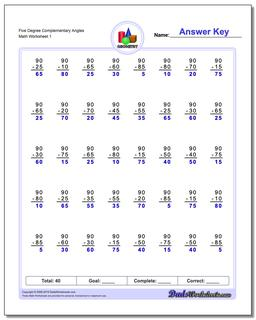# Math Worksheets: Basic Geometry: Basic Geometry: Five Degree Complementary Angles## Five Degree Complementary Angles

PropertyValue
DescriptionFive Degree Complementary Angles: Geometry worksheets with subtraction problems for finding complementary angles (Angles whose sum is 90 degrees).
Resource TypeWorksheet